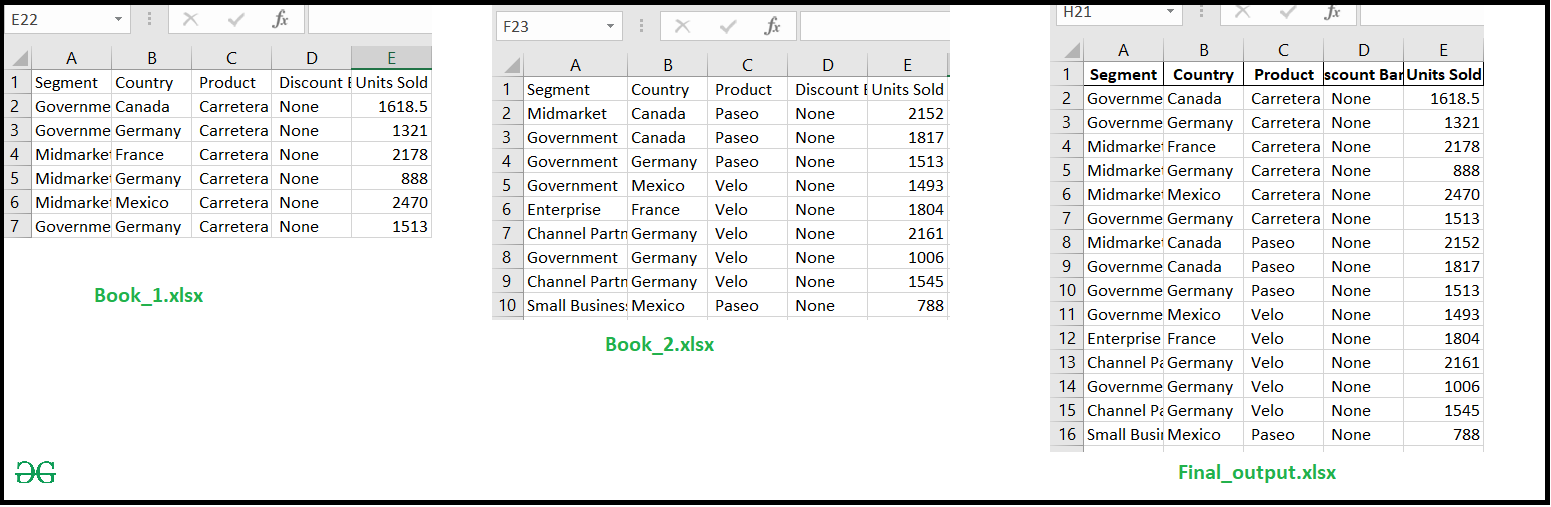Combine Multiple Excel Worksheets Into a Single Pandas Dataframe

• Last Updated : 05 Sep, 2020

Prerequisites: Working with excel files using Pandas

In these articles, we will discuss how to Import multiple excel sheet into a single DataFrame and save into a new excel file. Let’s suppose we have two Excel files with the same structure (Excel_1.xlsx, Excel_2.xlsx), then merge both of the sheets into a new Excel file.

Approach :

• Import-Module
• Read Excel file and store into a DataFrame
• Concat both DataFrame into a new DataFrame
• Export DataFrame into an Excel File with DataFrame.to_excel() function

Below is the implementation.

Python3

 # import moduleimport pandas as pd  # Read excel file# and store into a DataFramedf1 = pd.read_excel('excel_work\sample_data\Book_1.xlsx')df2 = pd.read_excel('excel_work\sample_data\Book_2.xlsx')  # concat both DataFrame into a single DataFramedf = pd.concat([df1, df2])  # Export Dataframe into Excel filedf.to_excel('final_output.xlsx', index=False)

Output :My Personal Notes arrow_drop_up